## Want to keep learning?

This content is taken from the RMIT University's online course, Online Business: Pricing for Success. Join the course to learn more.
4.6

## RMIT University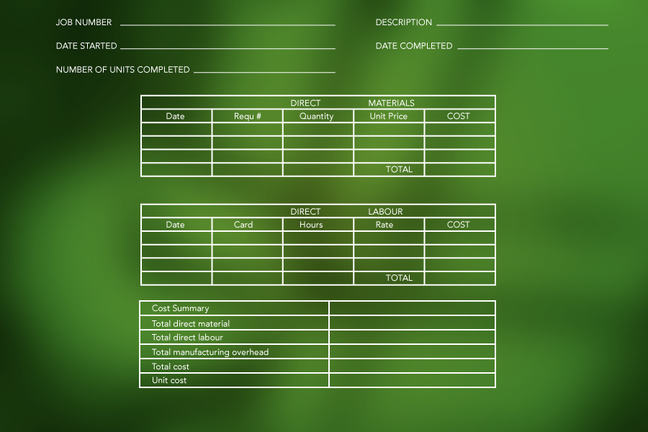Impact of fixed and variable costs in breakeven

# Impact of fixed and variable costs in breakeven

When calculating a breakeven amount, fixed costs and variable costs form part of the equation.

Fixed costs

Fixed costs are costs that remain the same regardless of the number of units you sell.

Costs such as rent of your offices, warehouse or other premises will still be incurred - even when you haven’t sold any products.

Let’s look at how to calculate fixed costs in relation to the units produced.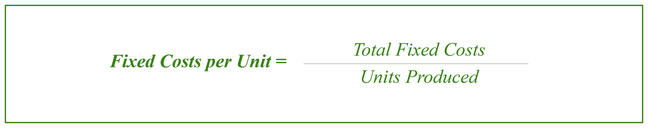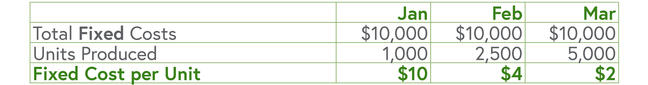This table shows that as you increase the number of units produced or sold, the fixed cost per unit decreases.

Variable costs

Variable costs are directly related to your products; if you source finished products, the variable costs would be the product cost plus delivery costs and any import duties; but If you manufacture, variable costs are the cost of materials used to produce the items, including labour costs.

Let’s look at an example, taking into account the fixed cost per units produced in the previous table; and now incorporate the variable cost per unit produced.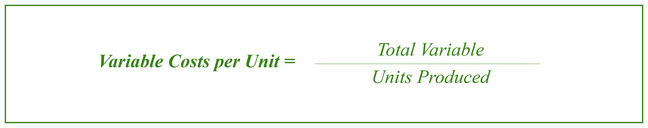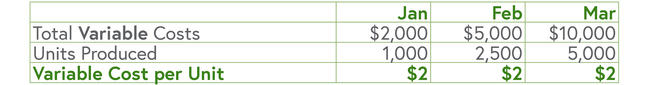This table shows that as production increases, the variable cost will also increase, however the variable cost per unit will remains the same.

Activity

Through the calculations above, we’ve established that the fixed costs per unit will move with production; but variable costs per unit do not move with production.

What would make the variable cost per unit move with production?